nextnano.com  nextnano³  Download | Search | Copyright | Publications  * password protected nextnano³ software3D artificial QD crystal

# nextnano3 - Tutorial

## Artificial quantum dot crystal - Superlattice dispersion (minibands)

Author: Stefan Birner

If you want to obtain the input files that are used within this tutorial, please check if you can find them in the installation directory.
If you cannot find them, please submit a Support Ticket.
```-> 3DSuperlatticeDispersion_ArtificialQDCrystal_cubic.in -> 3DSuperlatticeDispersion_ArtificialQDCrystal_tetragonal_025nmgrid_PRB.in```

## Artificial quantum dot crystal - Superlattice dispersion (minibands) of cubic QDs

`-> 3DSuperlatticeDispersion_ArtificialQDCrystal_cubic.in`

This tutorial is based on the following paper:

`[Balandin]`
Miniband formation in a quantum dot crystal
O.L. Lazarenkova, A.A. Balandin
Journal of Applied Physics 89, 5509 (2001)

We calculate the superlattice energy dispersion for an artificial crystal consisting of cubic QDs.

• The QD has a cubic shape with Lx = Ly = Lz = 6.5 nm.
• The QDs are separated by a distance of Hx = Hy = Hz = 1.5 nm.
• superlattice period: dx = dy = dz = 8 nm      (di = Li + Hi)
• We only model one QD and assume periodic boundary conditions along the x, y and z direction.
• We solve the single-band Schrödinger equation for the valence band only (heavy hole).
• The valence band offset is assumed to be VBO = 0.45 eV,
i.e. the valence band edge of the QD is at +0.45 eV, the valence band edge of the barrier is at 0 eV.
• QD material: Ge, barrier material: Si
• effective heavy hole masses (isotropic):
QD: 0.28 m0 (Ge), barrier: 0.49 m0 (Si)
• grid spacing: 0.5 nm in x, y and z direction, i.e. 16 grid points in each direction.
size of Schrödinger matrix to be solved: 16 * 16 * 16 = 4096
(60 eigenvalues are calculated)

Required superlattice options

``` \$numeric-control   ...  !superlattice-option = 100-only ! ```for dispersion along  direction in superlattice Brillouin zone, i.e. from Gamma to X```   superlattice-option = 110-only ! ```for dispersion along  direction in superlattice Brillouin zone, i.e. from Gamma to K```  !superlattice-option = 111-only ! ```for dispersion along  direction in superlattice Brillouin zone, i.e. from Gamma to L```  ```

``` \$quantum-model-holes   ...   model-name                     = effective-mass !   number-of-eigenvalues-per-band = 60             ! ```how many eigenenergies have to be calculated in each band```   boundary-condition-100         = periodic       ! ```periodic boundary conditions along x direction```   boundary-condition-010         = periodic       ! ```periodic boundary conditions along y direction```   boundary-condition-001         = periodic       ! ```periodic boundary conditions along z direction```  !num-ks-100                     = 1              ! ```i.e. no superlattice```   num-ks-100                     = 11             ! ```number of superlattice vectors along x direction```  !num-ks-010                     = 1              ! ```i.e. no superlattice```   num-ks-010                     = 11             ! ```number of superlattice vectors along x direction```  !num-ks-001                     = 1              ! ```i.e. no superlattice```   num-ks-001                     = 11             ! ```number of superlattice vectors along x direction```  ```

## Dispersion relation along  direction

```  superlattice-option = 100-only ! ```for dispersion along  direction in superlattice Brillouin zone, i.e. from Gamma to X

The following figure shows the calculated dispersion relation along the  direction.
The figure agrees very well with Fig. 2(a) of the paper by``` [Balandin] ```in the energy region where the confinement inside the QD is strong.
For the higher lying states inside the QD and above the QD, our results differ because we use the correct 3D QD confinement potential whereas``` [Balandin] ```approximated the potential landscape with an analytical ansatz that allows for the separation of the x, y and z variables. (This ansatz is only justified for states confined deep inside the QD.)
The right part of the figure shows schematically the valence band edge of the QD with the energy levels of the single, uncoupled QD.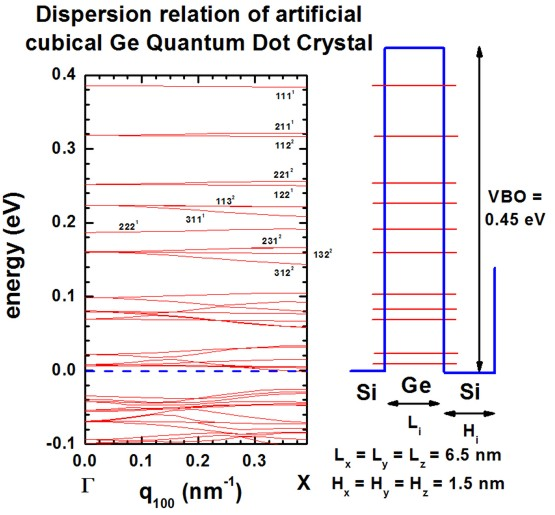In the figure, the eigenstates are labeled with the quantum numbers nxnynz, e.g. 111.
The superscript refers to their degeneracy.
At KSL=0, the degeneracy is higher than at nonzero KSL vectors where the symmetry in the Brillouin zone is lower.
(The superlattice vector "KSL" is often denoted as "q".)

## Dispersion relation along  and  direction

```  superlattice-option = 110-only ! ```for dispersion along  direction in superlattice Brillouin zone, i.e. from Gamma to K```  !superlattice-option = 111-only ! ```for dispersion along  direction in superlattice Brillouin zone, i.e. from Gamma to L```  ```

The following figure shows the calculated dispersion relations along the  and  directions.
The agreement to Fig. 2(b) and 2(c) of` [Balandin] `is again very good for the states that lie deep inside the QD (see also comments above).
Note that the eigenstates along the  direction show a higher degree of degeneracy throughout the superlattice Brillouin zone as compared to  and .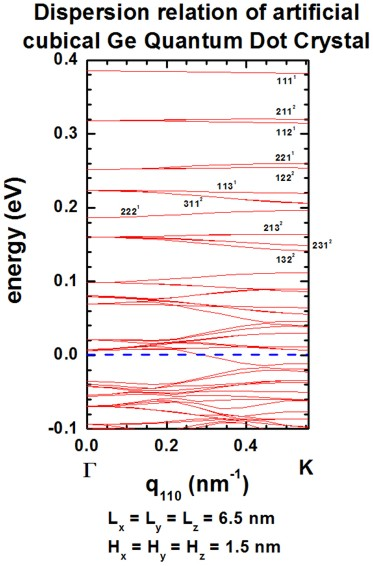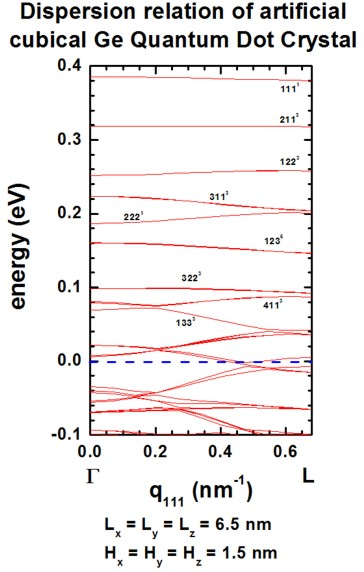Both, the QD itself and the QD superlattice have the same cubic symmetry in this example. Thus the degeneracy of the 123 (incl. permutations) energy band is sixfold throughout the Brillouin zone along the  directions (as shown in right figure).

## Output

The output that was plotted in these figures can be found in this file:
```Schroedinger_1band/sg_dispSL3D_hl_qc001_sg001_deg001_evmin001_evmax060.dat ```This file contains information about the superlattice vector KSL (in units of [pi/Li] and [1/Angstrom]), and the corresponding eigenvalues.```  norm[pi/L] norm[1/AA] k_x[pi/L_x] k_x[1/AA] k_y[pi/L_y] k_y[1/AA] k_z[pi/L_z] k_z[1/AA] 1 2 3 ... 60  ...        ...        ...         ...       ...         ...       ...         ...       . . . ... .```

## Artificial quantum dot crystal - Superlattice dispersion (minibands) of tetragonal QDs

```-> 3DSuperlatticeDispersion_ArtificialQDCrystal_tetragonal_025nmgrid_PRB.in```

See also the following paper for similar calculations, especially with respect to comparing the numercial vs. the analytical (separation of variables) results.

`[Balandin2]`
Electron and phonon energy spectra in a three-dimensional regimented quantum dot superlattice},
O.L. Lazarenkova, A.A. Balandin
Physical Review B 66, 245319 (2002)

We calculate the superlattice energy dispersion for an artificial crystal consisting of tetragonal QDs.

• The QD has an tetragonal shape with Lx = Ly = 5 nm and Lz = 2.5 nm.
• The QDs are separated by a distance of Hx = Hy = 2.5 nm and Hz = 1.25 nm.
• superlattice period: dx = dy = 7.5 nm and dz = 3.75 nm      (di = Li + Hi)
• grid spacing: 0.25 nm in x, y and z direction, i.e. 30 grid points in x and y direction, 15 grid points in z direction.
size of Schrödinger matrix to be solved: 30 * 30 * 15 = 13500
• All other assumptions are the same as for the above cubic QD example.

The following figures show the dispersion along the ,  and  directions.
All results are in very good agreement to Fig. 4 of` [Balandin]`.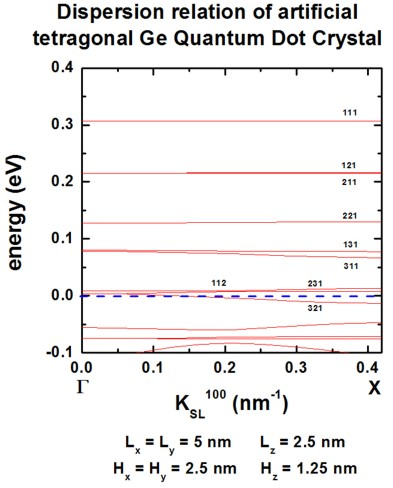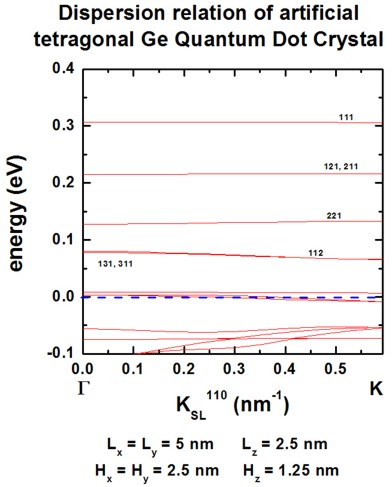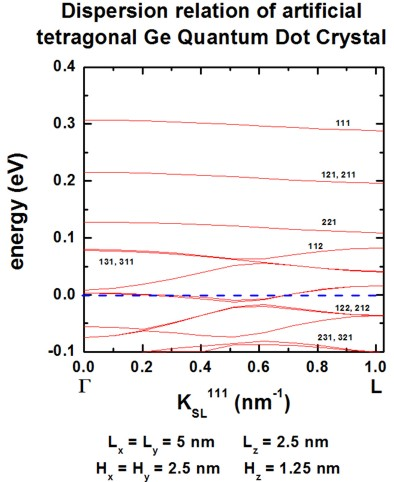See also this tutorial for one-dimensional superlattices: Dispersion in infinite superlattices: Minibands